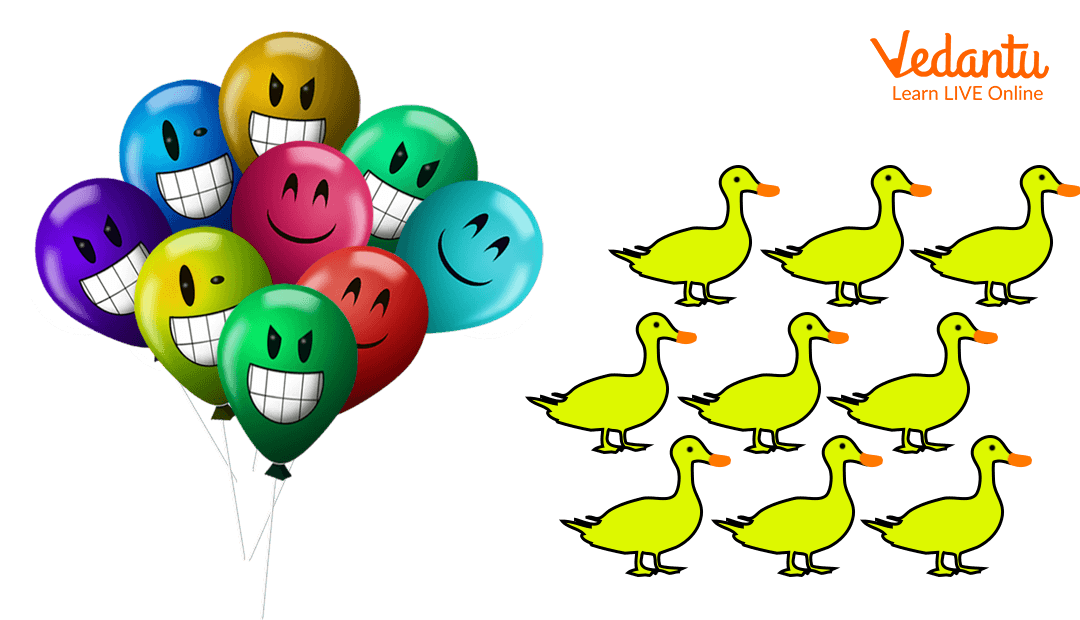Courses
Courses for Kids
Free study material
Free LIVE classes
More

# An Introduction to Number 9LIVE
Join Vedantu’s FREE Mastercalss

## Learn and Sing with Number 9 - An introduction to Number 9

Do you know about the amazing number 9? If no, then worry not for we are here to introduce you to this number 9. This number is one of the crucial numbers in the number system. The number comes before 10 and after 8.

This was just a brief introduction to the number 9. We will be discussing what exactly is number 9, the formation or the origin of the number 9, learning the count to number 9, and also singing with the number 9. Thus, with this let us first start with ‘what is 9’?

## Know about the Number 9

As we have mentioned in the previous paragraph, the number 9 is an important number in the mathematics number system. The number 9 helps us in the calculations or counting of the data. In maths, the number 9 represents the quantity or value of 9. In word form, the number 9 is written as - Nine.

## Your Marks without the Number 9!

In your school, you must have scored 90+ in many subjects. Imagine how difficult it would be if 9 as a number did not exist at all! You might have never gotten 90+, that would be bad. So, we see how the number 9 is helpful to us.

## Divine 9 - The Origin of the Number 9

Number 9 originated in the Indian Subcontinent in the early 3000 B.C. According to the Hindus, this number is denoted as a complete, perfected, and divine number as it completes a whole cycle of the decimal system.

## Let us Count to Number 9

After we have studied in-depth about the number and known its origin, it is time to count to the number 9. Let us begin:

0, 1, 2, 3, 4, 5, 6, 7, 8, and 9!

Do you see that 9 completes the number system? Well, after 9 comes 10 but that is only a mere addition of 1 and 0 making it to number 10.

## Let’s Count the Number of Little Ila’s Raising FingersCounting to the number 9

Little Ila is raising her fingers and counting to the number 9, good progress Ila!

Now, let us see if we can count like Ila.

Count the total number of balloons and ducks in the picture given below:Counting to the number 9

In the picture above, we see 9 balloons and 9 ducks.

Well done in counting! Now as a bonus point let us sing to number 9.

Let us sing!

Nine coloured balloons flying in the air,

Flip-flop, flip-flop they dance in a line.

So, count them on from one to nine!

## Did You Know?

1. Nine is a natural number in the number system.

2. This is an odd number.

3. 9 is also a composite number (not a prime number).

4. Nine is even the sum of the first three consecutive odd numbers in the number system: 1, 3, and 5 ( 1 + 3 + 5 = 9).

## Fun Ways to Teach Number 9 to Your Kids

This part of the content is dedicated to the parents and the teachers who are trying to teach Number 9 to their kids or students.

One of the effective and fun ways to learn numbers is by counting them. So, make the children count 9 colours or vegetables. Also, make them spell out the number 9. These exercises are to be done once they are fluent in the number system at least from 1 to 10.

To make the learning more interesting, share some amazing facts about the number 9 like -

• There are 9 players in a baseball team.

• The ninth month of the year, which is September is the only month whose the number of letters in the name is equal to 9. The ninth month, nine letters. Interesting right?

This was all about the Number 9. We hope you are now well introduced to this number. As you grow up, you will learn calculation with this amazing number 9. Till then keep practising counting to the number 9.

Last updated date: 21st Sep 2023
Total views: 136.2k
Views today: 3.36k

## FAQs on An Introduction to Number 9

1. Why is 9 known as a composite number?

### Nine is not a prime number and thus this is a composite number. Composite Numbers are those numbers that are divisible by more than two factors.  1 x 9 = 9 and also 3 x 3 = 9 thus, it is a composite number.

2. What does the number 9 represent in numerology?

In the study of numerology, the number 9 represents ‘completion’. Number 9 is the highest and the last of the single-digit numbers.